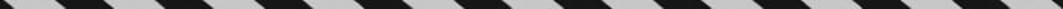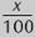﻿ ﻿PERCENTS - Fractions, Decimals, and Percents - TOPICS IN ARITHMETIC - SAT SUBJECT TEST MATH LEVEL 1

## TOPICS IN ARITHMETIC## CHAPTER 3Fractions, Decimals, and Percents### PERCENTS

As mentioned previously, the word percent means hundredth. We use the symbol % to express the word “percent.” For example, “23 percent” means “23 hundredths” and can be written with a % symbol, as a fraction, or as a decimal:Recall from TACTIC B2 that to take a percent of a number, you multiply. Since many calculators don”t have a % key, you first have to convert the percent to a decimal or fraction.

Remember

A percent is just a fraction whose denominator is 100.

Key Fact B11

To convert a percent to a decimal, drop the % symbol and move the decimal point two places to the left, adding 0”s if necessary. (Remember that we consider a whole number to have a decimal point to the right of it.)

To convert a percent to a fraction, drop the % symbol, write the number over 100, and reduce.Consider the three problems in the following example.

EXAMPLE 11:

I. What is 35% of 160?

II. 54 is 45% of what number?

III. 77 is what percent of 140?

You can easily solve each of these problems using your calculator if you first set them up properly. In each case, there is one unknown. Call the unknown x. Translate each sentence, replacing “is” by “ = ” and the unknown by x.I. x = 35% of 160x = (0.35)(160) = 56

II. 54 = 45% of x54 = 0.45x× = 540.45 = 120

III.Remember

Although 35% can be written as a fractionor a decimal (0.35), x% can only be written as.

Many students have been taught to solve problems such as these by writing the proportion. To use this method, think of “is,” “of,” and “percent” as variables. In each percent problem, you are given two of the variables and need to find the third, which you label x. Of course, you then solve such equations by cross multiplying.

In Example 12, each of the three problems in Example 11 is solved by using theproportion.

EXAMPLE 12:

I. What is 35% of 160? (Let x = the “is” number.)II. 54 is 45% of what number? (Let x = the “of ” number.)III. 77 is what % of 140? (Let x = the percent.)﻿